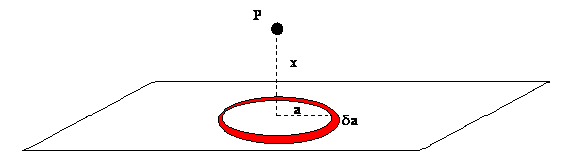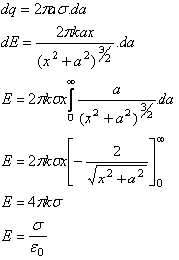# why the electric field due to a plane sheet of charge and electric field near a charged conducting surface?why they r different?

12 years ago

Let us first consider the electric field on the axis of a uniformly charged ring - you will see why this is relevant soon. This situation is shown in the diagram:We know that the net electric field will point towards the centre of the ring, since all vertical components will cancel out due to the symmetry of the shape. This means we need only consider the components in the direction of the ring. First we will consider the force on particle P due to the red element highlighted. By Coulombs law we know that the contribution to the field will be:Since all the terms are constant this means that the total electric field due to the ring will be:Now we will consider the problem of the infinite sheet. We think of the sheet as being composed of an infinite number of rings. We will let the charge per unit area equal sigma:All the best.

Win exciting gifts by answering the questions on Discussion Forum. So help discuss any query on askiitians forum and become an Elite Expert League askiitian.